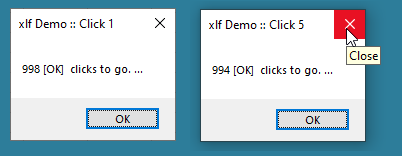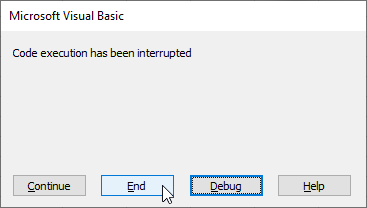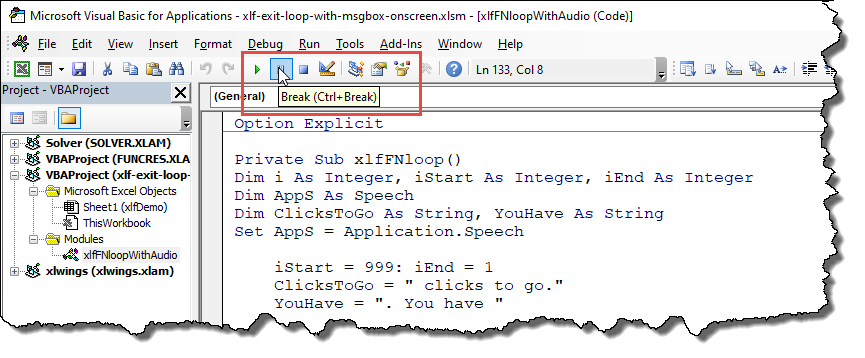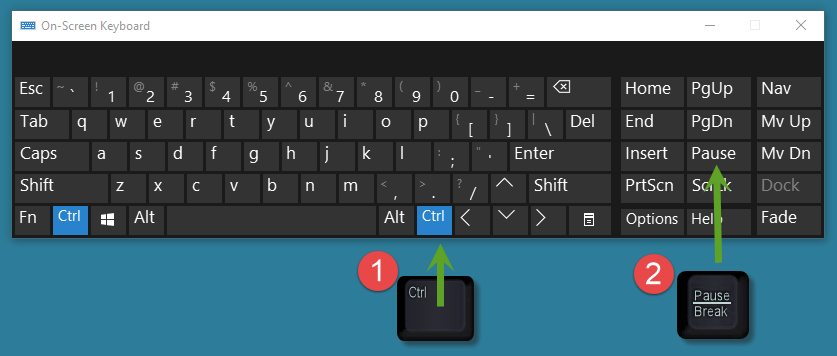# Break out of a Loop while Message Box is active

## 0. Quick guide - how to [Close] a message box when part of a loop

Suppose that a message box (MsgBox) is part of the statement structure in a For...Next loop. When clicked, the Close button in figure 1 has the same effect as the OK button. It simply continues the loop sequence.Fig 1: Simple message box demo with 999 click sequence - clicking the Close button [X] will only continue the sequence

### How do you exit early?

See the following indicators below for three possible techniques.

Ctrl + Break To break out of a loop, the conventional technique is the keyboard Ctrl + Break combination.

Ctrl + Break will display the dialog box in figure 2. Click [End] to exit loop, or [Debug] to see the code at the current Step Point in the VBE module window.Fig 2: Code execution interrupter dialog - activated by the Ctrl + Break keyboard combination.

Many laptop devices do not have a Break|Pause key creating a problem with the Ctrl + Break combination. The VBE has a Break control (figure 2), but this is not available because the message box is modal. This means the user must respond to the message box before continuing in the current application and is unable to interact with the VBE.Fig 3: VBE controls - the Break (Ctrl + Break) is not available when message box is on screen

## 1. Alternatives to Ctrl + Break

On-screen keyboard An on-screen keyboard available in Windows. Search for OSK (On-Screen Keyboard), or the menu sequence Windows > Settings > Ease of Access > Keyboard > Use the ON-Screen Keyboard.

From the OSK:

1. Click Ctrl
2. Then Click Pause
3. This will display the figure 2 dialog boxFig 3: On-Screen keyboard - click [Ctrl] then [Pause] to interrupt the current VBA procedure

Watch the video for a the message box click sequence, and examples of physical keyboard and on-screen keyboard interrupt events

Another techniques is to add a Cancel button to the message box. When added, the Close button is also functional

## 2. VBA code

### 2.1 Message box with audio - in For...Next loop

In code 1 the For...Next loop runs from lines 15 to 32, with a simple decrementing counter and audio channel.

Code 1: MsgBox xlfFNloop - xlfFNloopWithAudio code module

Private Sub xlfFNloop()
Dim i As Integer, iStart As Integer, iEnd As Integer
Dim AppS As Speech
Dim ClicksToGo As String, YouHave As String
Set AppS = Application.Speech

iStart = 999: iEnd = 1
ClicksToGo = " clicks to go."
YouHave = ". You have "

For i = iStart To iEnd Step -1
If i - 1 = 1 Then
ClicksToGo = " click to go"
ElseIf i - 1 = 0 Then
YouHave = ". This is the final click"
ClicksToGo = ""
End If

' Speech ============
AppS.Speak ("Click " & NumberToWords(iStart - i + 1) & _
YouHave & NumberToWords(i - 1) & ClicksToGo), _
SpeakAsync:=True, _
Purge:=True

' Message box =======
MsgBox Prompt:=(i - 1 & " [OK] " & ClicksToGo & " ..."), _
Title:="xlf Demo :: Click " & iStart - i + 1
Next i

End Sub



### 2.2 VBA - number to words

Converting number to words is achieved by the three function in code 2. NumberToWords at line 40, GetTens at line 68, and GetDigit at line 114.

Code 2: Private functions NumberToWords and associated code -xlfFNloopWithAudio code module
Private Function NumberToWords(ByVal Number As String) As String
' Numbers 1 to 999
Dim Temp As String

Number = Right("000" & Number, 3)

' 100 to 999
If Left(Number, 1) <> "0" Then
Temp = GetDigit(Left(Number, 1)) & " Hundred and "
End If

If Mid(Number, 2, 1) <> "0" Then
Temp = Temp & GetTens(Mid(Number, 2))   ' 10 to 99
Else
Temp = Temp & GetDigit(Mid(Number, 3))  ' 1 to 9
End If

NumberToWords = Temp

End Function

Private Sub TestNumberToWords()
Dim Ans As String
Ans = NumberToWords(999)
Stop
End Sub

Private Function GetTens(Tens As Integer) As String
' Numbers 10 to 99
Dim Temp As String

If Val(Left(Tens, 1)) = 1 Then ' value between 10 to 19
Select Case Val(Tens)
Case 10: Temp = "Ten"
Case 11: Temp = "Eleven"
Case 12: Temp = "Twelve"
Case 13: Temp = "Thirteen"
Case 14: Temp = "Fourteen"
Case 15: Temp = "Fifteen"
Case 16: Temp = "Sixteen"
Case 17: Temp = "Seventeen"
Case 18: Temp = "Eighteen"
Case 19: Temp = "Nineteen"
Case Else  ' do nothing
End Select
Else ' value between 20 to 90 step 10
Select Case Val(Left(Tens, 1))
Case 2: Temp = "Twenty "
Case 3: Temp = "Thirty "
Case 4: Temp = "Forty "
Case 5: Temp = "Fifty "
Case 6: Temp = "Sixty "
Case 7: Temp = "Seventy "
Case 8: Temp = "Eighty "
Case 9: Temp = "Ninety "
Case Else ' do nothing
End Select

' combine tens and digit
Temp = Temp & GetDigit(Right(Tens, 1))
End If

GetTens = Temp

End Function

Private Sub TestGetTens()
Dim Ans As String
Ans = GetTens(99)
Stop
End Sub

Private Function GetDigit(Digit As Integer) As String
' Digits 1 to 9
Select Case Val(Digit)
Case 0: GetDigit = ""
Case 1: GetDigit = "One"
Case 2: GetDigit = "Two"
Case 3: GetDigit = "Three"
Case 4: GetDigit = "Four"
Case 5: GetDigit = "Five"
Case 6: GetDigit = "Six"
Case 7: GetDigit = "Seven"
Case 8: GetDigit = "Eight"
Case 9: GetDigit = "Nine"
Case Else  ' do nothing
End Select
End Function

Private Sub TestGetDigit()
Dim Ans As String
Ans = GetDigit(3)
Stop
End Sub



### 2.3 WS controls for navigation

Code 3: Label control Question_Click - goto place mark on WS
Private Sub Question_Click()
Range("xlfDemo!BookMark1").Select
End Sub



### 2.4 Message box with OK and Cancel buttons

Add the button at line 171, then capture and process response at lines 173 and 174.

Code 3: Label control xlfFNloopExit - xlfFNloopWithAudio code module
Public Sub xlfFNloopExit()
Dim i As Integer, iStart As Integer, iEnd As Integer
Dim AppS As Speech
Dim ClicksToGo As String, YouHave As String
Dim Response As Integer
Set AppS = Application.Speech

iStart = 999: iEnd = 1
ClicksToGo = " clicks to go."
YouHave = ". You have "

For i = iStart To iEnd Step -1
If i - 1 = 1 Then
ClicksToGo = " click to go"
ElseIf i - 1 = 0 Then
YouHave = ". This is the final click"
ClicksToGo = ""
End If

' Speech ============
AppS.Speak ("Click " & NumberToWords(iStart - i + 1) & _
YouHave & NumberToWords(i - 1) & ClicksToGo), _
SpeakAsync:=True, _
Purge:=True

' Message box =======
Response = MsgBox(Prompt:=(i - 1 & " [OK] " & ClicksToGo & " ..."), _
Title:="xlf Demo :: Click " & iStart - i + 1, _
Buttons:=vbOKCancel)
If Response = vbCancel Then Exit For
Next i

End Sub